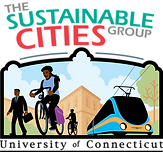###### due Wed April 17##### Question 1
###### ​

Estimate the design hour volume in the design direction for Storrs Road (SR 195) given that the

AADT = 10,000, and the directional split is 0.60:0.40.

Use the 60th hour as the design hour and estimate the relationship between AADT and design hour using the AASHTO chart from the class notes.

Assume that the line for the average conditions applies to Storrs Road.

Determine the number of lanes that will be needed in each direction to carry this traffic at a LOS E.

The maximum volume for each level of service on this type of road is given below.

LOS C 290, LOS D 780, LOS E 880, LOS F >880

##### Question 2
###### ​

A curve has external angle of 20 degrees, a radius of 200 meters and the BC is at station 12+00 (1200 meters from the start of the section). Calculate

1.     the station of the EC

2.     the station of the PI

3.     the degree of curvature (you need to convert units to feet)

4.     the middle ordinate (M)

##### Question 3
###### ​

A curve of radius 1000 ft is used at a location with an external angle (DELTA) of 30o.  Calculate the length of the curve needed.

Calculate the length of each curve segment if a spiral transition curve is used with the circular curve.  The k-value for the spiral curve is 3 degrees per 100 feet.

Describe the reasons for using a spiral curve.